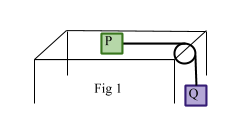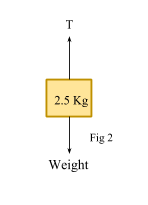# Using Newton’s Law

## Summary

• We have already studied Newton’s Three Laws of Motion in our previous article.
• Recall F = ma from Newton’s Second Law of motion where F is the Force on the moving body, mis the mass and ais the acceleration of the moving body.
• When two particles are connected with a string and the string passes over a fixed pulley, then we assume a few restrictions that we must remember when solving such problems:

1)    We assume that the string is light.
2)    The string is inextensible
3)    Pulley is smooth
4)    Pulley is fixed
5)    Air resistance is not taken into account.
6)    Forces on each particle are considered separately and F = ma is applied to each particle.
7)    We consider the moving bodies as particles.

To revise let’s recall from the article “Newton’s Laws of Motion”, the three laws which were defined as:

• Newton’s First Law of Motion: Every body continues in a state of rest or if uniform motion in a straight line unless it is acted upon by an external force and is compelled to change its state.
• Newton’s Second Law of Motion: The rate of change of momentum of a body is directly proportional to the force acting and takes place in the direction of that force.  F = ma
• Newton’s Third Law of Motion: To every action, there is always an equal and opposite reaction.

Let’s now move on to study motion of two particles connected with a string, when the string passes over a fixed pulley, we usually just assume the following restrictions:

i) The string is light:  We assume that the string is light because If the string is heavy then the tension will vary from point to point depending upon the weight/unit length.

ii) The string is inextensible: If the string is inextensible, then tension will depend upon its extension at a given point. Hooke’s law will then be used and hence tension will vary from point to point.

iii) Pulley is smooth: If the pulley is smooth, then the tension will remain the same on the two sides of the string. If the pulley is rough, then the tension in the string will depend upon the coefficient of friction.

iv) Pulley is fixed: If the pulley is not fixed then rotational motion of the pulley will come into play and we will have different tensions in the parts of the string on either sides of the pulley.

Thus in our ideal mathematical model:

• String is light and inextensible
• Pulley is fixed and smooth
• Moving bodies are considered as particles
• Air resistance is not taken into account
• Forces on each particle are considered separately and F = ma is applied to each particle

#### Example #1

Q. Two particles P and Q of mass 1.7 Kg and 0.2 Kg are connected by a light inextensible string as shown in Fig 1. P is held on a smooth horizontal table with the string taut and passing over a smooth fixed pulley fixed at the edge of a table. Q is at rest and P is released. Find the acceleration of the particles and the tension in the string.Solution:

For the particle Q, the resultant downward force is  = 3 – T

Apply F = ma, where a is the common acceleration$3\quad -\quad T\quad =\quad 0.3a\quad \quad \longrightarrow \quad (1)$

For particle P$T\quad =\quad 1.7a\quad \quad \longrightarrow \quad (2)$

Adding equation (1) and equation (2), we get

3 = 0.3a + 1.7a$a\quad =\quad \frac { 3 }{ 2 } { m }/{ { s }^{ 2 } }$

Substituting the value of acceleration in equation (2)$T\quad =\quad 1.7(\frac { 3 }{ 2 } )$

T = 2.55 N     Ans

#### Example #2

Q. A bucket of mass 2.5 kg is pulled upwards by a rope. It accelerates upwards at 2.2$m{ s }^{ -2 }$.Find the tension in the rope.

Solution:

We know acceleration here is due to gravity g=9.8$m{ s }^{ -2 }$.

Weight = m g
= 2.5 (9.8)
= 24.5  N

Resultant Force in upward direction is  = (T – 24.5) N

Therefore:

Resultant Force = m a

Where a = 2.2$m{ s }^{ -2 }$

(T – 24.5) = 2.5 (2.2)

T – 24.5 = 5.5

T = 5.5 + 24.5

T = 30 N      Ans